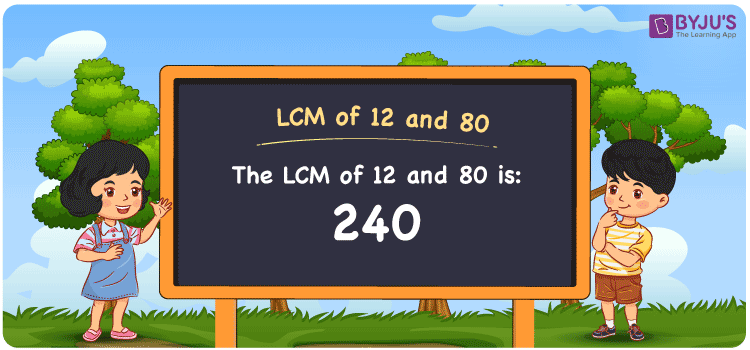# LCM of 12 and 80

LCM of 12 and 80 is 240. LCM, known as Least Common Multiple or Lowest Common Multiple, is the smallest number that is divisible by the given numbers. Consider the example for finding the LCM of 12 and 80. The answer is 240. 240 is divisible by both 12 and 80. Even 480 is divisible by 12 and 80, however it is not the LCM for 12 and 80. The smaller number than 72 is 240 which is divisible by both 12 and 80. Hence 240 is the Least Common Multiple for 12 and 80. You can use the Prime Factorization and Division method to find the LCM.

## What is LCM of 12 and 80

The Least Common Multiple or Lowest Common Multiple of 12 and 80 is 240.## How to Find LCM of 12 and 80?

LCM of 12 and 80 can be determined using three methods:

• Prime Factorisation
• Division method
• Listing the multiples

### LCM of 12 and 80 Using Prime Factorisation Method

In the Prime Factorization method, the given numbers 12 and 80, it is

12 = 2 × 2 × 3

80 = 2 × 2 × 2 × 2 × 5

2 × 2 is the common factor for both 12 and 80, and the other factors are multiplied to get the LCM.

Hence, LCM (12, 80) = 2 × 2 × 2 × 2 × 3 × 5 = 240

### LCM of 12 and 80 Using Division Method

In the Division Method, the given set of numbers 12 and 80 are divided together by common factors. The division is completed with other factors until the remainders become one. The product of common and uncommon factors of 12 and 80 to get the LCM.

 2 12 80 2 6 40 2 3 20 2 3 10 3 3 5 5 1 5 × 1 1

Hence, LCM (12, 80) = 2 × 2 × 2 × 2 × 3 × 5 = 240

### LCM of 12 and 80 Using Listing the Multiples

By listing all the multiples of 12 and 80, we can identify the LCM. Below is the list of multiples for 12 and 80;

Multiples of 12

12, 24, 36, 48, 60, 72, 84, 96, 108, 120, 132, 144, 156, 168, 180, 192, 204, 216, 228, 240, 252, 264

Multiples of 80

80, 160, 240, 320, 400

LCM (12, 18) = 240

## Video Lesson on Applications of LCM## Solved Examples

What is the smallest number that is divisible by both 12 and 80?

Answer: 240 is the smallest number that is divisible by both 12 and 80.

What is the LCM for 2, 4, 12 and 80?

Answer: LCM for 2, 4, 12 and 80 is 240.

## Frequently Asked Questions on LCM of 12 and 80

### What is the LCM of 12 and 80?

The LCM of 12 and 80 is 240.

### Are LCM of 12 and 80 same as the LCM of 2 and 240?

Yes. LCM of 12 and 80 is 240 and LCM of 2 and 240 is also 240.

### Are LCM and LCD both the same for 12 and 80?

Yes. The Least Common Multiple and Least Common Divisor are both the same for 12 and 80, which is 240.

### What are the first 3 common multiples of 12 and 80?

The first three common multiples of 12 and 80 are 240, 480, and 720.

### Is the LCM of 6 and 12 the same as the LCM of 8 and 80?

No. LCM of 6 and 12 is 12, whereas the LCM of 8 and 80 is 80. They are not the same.